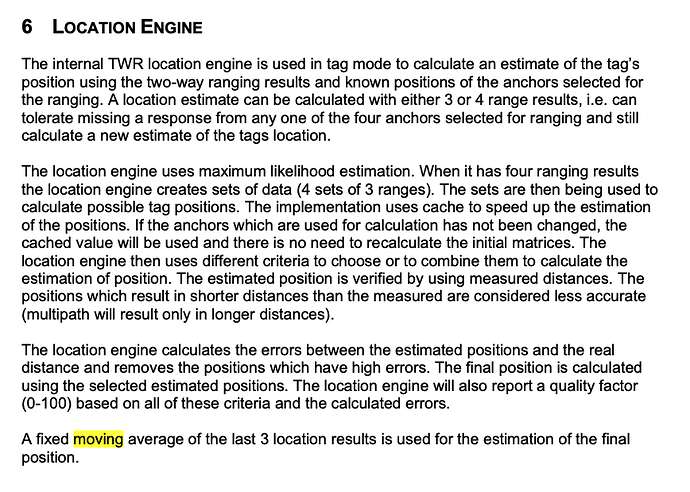# Location Engine AlgorithmCan anyone help me about the location engine aglorithm？(math process
I saw the document about location engine, and I knew that it uses maximum likelihood estimation.
I want to ask what it the probability distribution in this likelihood estimator?

I found someone said “In PANS SW (DWM1001/MDEK), algorithm uses 4 anchors (actually gets 4 ranges, and uses sets of 3 at a time, then gets average) to estimate tag’s position.”
So I feel confused…

1 Like

Hi @el_0813
it is a proprietary solution. But basically the location engine always uses only 3 ranges for position calculation (least square method). If there is only 3 anchors in range then it is one set of 3 ranges - it calculate only one solution. But if 4 anchor are in range then it uses all ranges variants and calculate multiple solution - once it is calculated then it pick up the most probably solution (be recalculation computed position to distances to anchors and compare them).

Cheers
JK

3 Likes